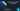# Dart Programming Language Tutorial Part 2 | Variables

August 28th, 2018
|

In this post we will look into various ways in which we can declare and use variable. We will also learn some syntax of dart and how to print values of variables. Let's dive right into it.

# The main function

Every dart program starts executing from a main function. What is a function? How do we use it? Weren't we going to learn about variables? Hold on, just bear with me for couple of posts till we will write all our code inside a wrapper. So open dartpad and let's start coding.

``````void main(){
// code starts here

// code ends here
}``````

For now just paste the above code snippet in your dartpad workspace, and we will write all our code between those two `code starts here` and ```code ends here``` lines.

# Declaration and Initialization of Variables

What is a variable? Well a variable is a container, which just holds some value in your program. Let's say you want to store the value of `pi` in your program, you need some place, a container to store that number `3.14`. That container is a variable.

To use a variable in your program you must declare and initialize it. So what is declaring and initializing a variable?

Declaring a variable is nothing but notifying everyone that a particular character or word will be used as a container to store something in the program. Initializing a variable is putting a initial value in that container i.e. giving a value to a variable for the first time. Let's look at some code.

``````    void main(){
// code starts here

int a = 3;

int b;
b = 4;

// code ends here;

}``````

In the code snippet above `int a = 3` is a variable declaration as well as initialization, a is declared that it will hold integer type of value, and is assigned a value of 3 for the first time. In the second case `int b;` is just a declaration where b is declared to be of integer type, and `b=3` is an initialization, since it is assigned a value for the first time.

# Different types of declaration in dart

Now we will look into different ways by which we can declare a variable in dart and their differences.

``````    void main(){
// code starts here

int a = 3;
var b = 3;
dynamic c = 3;

// code starts here

}``````

We have already looked into first one here, it declares a integer variable. We will look into other data types in greater detail in later posts, for now let's stick to integers. Second one is very similar to the first. It auto infers the data type from the initialization, so b will become an integer type automatically. Declaring local variables using var is recommended in dart. These two variable declaration are static declaration i.e. their type cannot be changed, we cannot put a string value into them later on in the program.

The third type is a interesting one, it is as the name suggests dynamic declaration. Even though the variable is assigned a integer value, we can put any type of value in the variable later on in the program.

# Printing Values

Now that we have three variables in our program, let's go ahead and print them.

``````    void mian(){
// code starts here

int a = 3;
var b = 3;
dynamic c = 3;

c = "stuart";

print(a);
print(b);
print(c);
}``````

Notice two things here, I changed the value of `c` to a string, I cannot do the same thing with `a` and `b` . Second, semicolons in dart are required. Next is a simple print statement to print the values of our variables to screen.